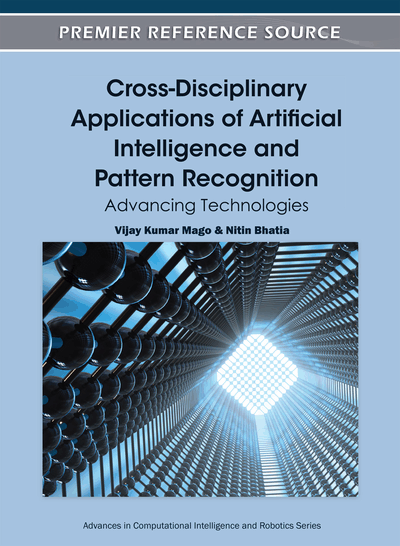# Classification with Axis-Aligned Rectangular Boundaries

Sung Hee Park (Stanford University, USA)
DOI: 10.4018/978-1-61350-429-1.ch019

## Abstract

This chapter presents a new method for binary classification that classifies input data into two regions separated by axis-aligned rectangular boundaries. Given the number of rectangular regions to use, this algorithm automatically finds the best boundaries that are determined concurrently. The formulation of the optimization problem involves minimizing the sum of minimum functions. To solve this problem, the author introduces underestimate of the minimum function with piecewise linear and convex envelope, which results in mixed integer and linear programming. The author shows several results of the algorithm and compare the effects of each term in the objective function. Finally, the chapter demonstrates that the method can be used in image capturing application to determine the optimal scheme that minimizes the total readout time of pixel data.
Chapter Preview
Top

## Background

Classification has been intensively studied in machine learning and pattern recognition (Jain, Duin & Mao, 2000). Researchers have developed tons of methods to tackle their problems and various approaches have evolved to handle specific applications. Here, we would like to mainly focus on the characteristics of the resulting decision boundaries that each classification technique provides. In some real world applications, users might have very specific constraints on the geometry of decision boundaries they will get in the end. Even more, users sometimes want to construct their boundaries to have certain shapes, such as circles, ellipses or polygons. However, most of classifiers do not explicitly focus on the geometry of results they provide. One main approach in classification is based on similarity matching. Popular methods such as template matching, the minimum distance classifier and k-nearest neighbor algorithm fall into this category. Although these methods usually results in piece-wise linear decision boundaries, the classifiers are implicitly determined and no control on the boundaries is provided to users. Another main approach is the probabilistic approach. The Bayes decision rule (Fukunaga, 1990) and a logistic classifier (Anderson, 1982) try to maximize a likelihood function to construct decision rules. Depending on which assumption they make on the distribution of data, they result in linear or quadratic decision boundaries, which we don't have any control on as the previous cases. The third category of classifiers is to explicitly determine decision boundaries by optimizing classification error costs. These methods allow direct handling of the geometry of the decision rules. However, many approaches such as Fisher's linear discriminant and the perceptron are limited to a linear case. Another popular approach in machine learning is to use support vector machines (Cortex & Vapnik, 1995). This class of methods is based on optimizing classification errors while maximizing the geometric margin to provide a maximum-margin hyperplane that separates the data. Even though this approach is effective for various applications, extension to nonlinear classification is not trivial. The kernel trick is applied to achieve nonlinear decision boundaries, but they are no longer explicitly handled in the original input space.

## Complete Chapter List

Search this Book:
Reset